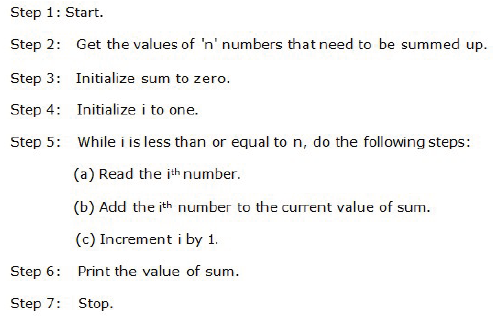#### Summation of a set of numbers

Problem Definition: Summation of a set of numbers.

Problem Analysis: Given a list of ‘n’ numbers, the algorithm should add up the ‘n’ numbers to find the sum of these values.

Solving by Example: Consider a list of 5 numbers.The algorithm takes these numbers as input and should produce the output as 47.

Algorithm Definition